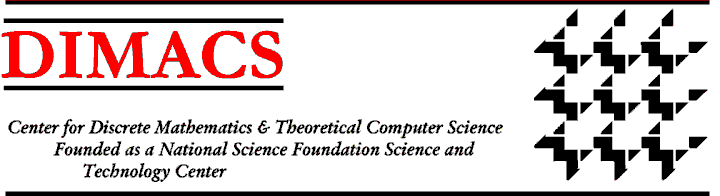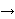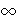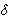### DIMACS/DIMATIA/Renyi Working Group on Extremal Combinatorics

Working Group Meetings:
First Meeting
Dates: April 10 - 16, 2003
Location: Alfred Renyi Institute, Budapest, Hungary
Second Meeting
Dates: October 18 - 20, 2004
Location: DIMACS Center, CoRE Building, Rutgers University, Piscataway, NJ
Third Meeting
Dates: July 30 - 31, 2005
Location: Prague, Czech Republic
Organizers:
Gyula Katona, Alfred Renyi Institute, ohkatona@renyi.hu

#### Working Group Agenda:

This working group will concentrate on two general topics, extremal graph theory and extremal problems arising from combinatorial search and testing.

Extremal graph theory ([9, 34]) deals with graphs satisfying specified constraints and optimizing some criterion. We plan to investigate jointly a number of specific questions of current research interest in this field, which has played a fundamental role in the history of graph theory.

The generalized matching problem is to find many vertex-disjoint copies of a fixed graph in a large graph. A corresponding extremal graph theory notion was developed for certain fixed graphs and very recently extended for all possible fixed graphs by Komlós . The working group will collaborate on identifying the precise extremal graphs in connection with this notion.

In general, we shall be interested in Turán-type extremal problems. Here, a family L of sample graphs is fixed and we consider various conditions on a graph on n vertices that does not contain any graph in L as a subgraph (not necessarily induced). The most famous such problem asks for the maximum number of edges ext(n,L) of a graph G on n vertices that does not have any subgraph in L. Turán solved this problem if L consists of the complete graph Kp+1 and asked the same question for other cases where L consists of a single graph. His goal was not so much to determine the extremal number of edges as to discover some important new phenomena by solving the problem for different cases. Surprisingly enough, some very simple cases of this problem remain open, e.g., the case of the cube Q8. Erdös and Simonovits  gave an upper bound for ext(n,Q8) that they conjectured to be sharp, but this conjecture remains unsettled and we will investigate it. Even open is the conjecture that ext(n,Q8)/n3/2. Simonovits  showed how some old methods can be used to solve new Turán-type extremal problems, for instance the determination of ext(n,P) where P is the Peterson graph. We shall try to make use of similar ideas. Let us say that a graph has property Bp,t if one cannot delete t-1 vertices from it to get a p-colorable graph. A generalization of the Turán-type problem is to find the maximum number of edges of a graph of n vertices that contains no graph of L and has property Bp,t. This type of question has been of interest since the work in . More generally of interest is the same question where property Bp,t is replaced by a condition that is called in the literature a ``chromatic condition'' (see ) and we shall study Turán-type problems under chromatic conditions.

The Peterson graph is a special case of the Kneser graph, which is defined as follows. Given a set A of a elements and an integer b < a/2, define the Kneser graph, Z(a,b) to be the graph whose vertices are the b-tuples of A and so that two of these b-tuples are joined by an edge if and only if they are disjoint. The Peterson graph is Z(5,2). The Kneser graph Z(a,b) can be colored in p = a-2b+2 colors. It was a longstanding conjecture of Kneser and a deep theorem of Lovász  (proved in a slightly simpler way by Bárány ) that this number actually gives the chromatic number. Recently, a unique combinatorial proof of Kneser's conjecture was presented by Ji\v{r}í Matou\v{s}ek (DIMATIA) at the DIMACS-DIMATIA workshop ``Homonolo'' in November 2000. Matou\v{s}ek derived this result from Tucker's combinatorial lemma on labeling the vertices of special triangulations of the octahedral ball. By specializing a proof of Tucker's lemma, he obtained a self-contained, purely combinatorial proof of Kneser's conjecture. It remains an open problem, which we plan to investigate, to find the minimum number p(a,b) of vertices that one can delete from the Kneser graph to get an a-2b+1-chromatic graph, and similarly for edges. Generalizations of Kneser's conjecture are still open for n-tuple colorings. In such a coloring, each vertex x is assigned a set C(x) of n colors in such a way that if x and y are adjacent, then C(x) and C(y) are disjoint. These colorings can be viewed as homomorphisms into Kneser graphs. We shall investigate an old conjecture of Stahl  giving the smallest number of colors in an n-tuple coloring of the Kneser graph (see also ). This problem relates to the work of the Working Group on Graph Coloring and Generalizations which will investigate generalizations of n-tuple colorings called set colorings. The Kneser graph is defined by subsets of a finite set. Many problems of extremal graph theory are interesting in the more general setting of extremal set theory or extremal hypergraph theory. (See for example [16, 17, 15, 24].) As time permits, the group will extend its work to these more general contexts.

An interesting problem is to find the minimum degree in a graph of n vertices that guarantees that the graph will have the Peterson graph as a subgraph. More generally, we ask for the maximum value dex(n,L) of the minimum degree>(G) of a graph G of n vertices that has no graph in family L as a subgraph. It is easy to see that dex(n,L) < (2/n)ext(n,L). As observed in , the upper bound is almost achieved in many interesting cases, and we shall try to identify conditions under which this is true.

In approaching problems in extremal graph theory, two of the main tools we shall use are the so-called Regularity Lemma of Szemerédi and the Blow-up Lemma that was developed by Komlós, Sárközy and Szemerédi . For surveys about these tools, see [27, 30].

This working group will also be concerned with several classes of combinatorial search and testing problems. In one such problem, a sequential test procedure, optimal with respect to an appropriate performance criterion, is to be designed to identify a single item from among a set of items, and the single item of interest is ``distinguished'' in some specific way recognizable by the tests. For example, we may be performing diagnostic tests to identify a faulty component within a system of components. If the system is monitored continuously for failure, it is reasonable to assume that there is a single faulty component present at the instant of system failure. Katona [25, 26] has investigated the specific variant of this problem in which there are constraints on the maximum number of items which can be included in any test, a situation corresponding to the case that the diagnostic device has some physical capacity associated with it. He has also investigated various models in which test results can be unreliable, obtaining (for both reliable and unreliable frameworks) bounds on the maximum number of tests required given that the goal is to minimize this quantity. We shall investigate a number of variants of the search problem, such as the case in which there are multiple diagnostic devices operating in parallel, and when we focus on the performance criterion of minimum average number of tests under a probability model imposed on the individual items for being the single distinguished one. Work of Abrahams  is relevant here. We plan to work on performance bounds for minimum-average-number-of-tests problems in which there are constraints on the individual test sizes. We will also work on a number of other problem variants, both for reliable and unreliable test models, particularly variants involving linearly and partially ordered constraints on the items.

The working group will also investigate the related theory and algorithms of combinatorial group testing (see ), with emphasis on connections to coding theory and combinatorial design. These topics are also of interest to the Working Group on Algebraic and Geometric Methods in Combinatorics. To identify all positive cases in a large population of items, group testing proceeds by grouping the items into subsets, testing if a subset contains at least one positive item, and then identifying all positive items through iteration of group tests. The theory of group testing arose via testing millions of World War II military draftees for syphilis  and it is very relevant to schemes for large-scale blood testing for viruses such as HIV. Group testing also arises in connection with the mapping of genomes (see, e.g., [5, 6, 10, 19, 32]). Here, we have a long list of molecular sequences, form a library of subsequences (clones), and test whether or not a particular sequence (a probe) appears in the library by testing to see which clones it appears in. Because clone libraries can be huge, we do this by pooling the clones into groups. Group testing is also relevant to the identification of defective products and has found application in satellite communications (see ). We will investigate these various applications of group testing. Among the specific questions we will explore are questions dealing with two-stage group testing. Here, the goal of the first stage is to identify a small subset of the items being tested that is sure to contain all the positive items. Some preliminary work in this area is contained in the papers [8, 11, 21] and we shall investigate it further, with emphasis on the problem of finding designs that achieve some of the bounds obtained in .

We shall also investigate problems involving testing properties of evolving objects. These problems can be formulated as determining whether a function f has property P or is-far from any function with property P. A property testing algorithm is given a sample of the value of f on instances drawn according to some distribution, and, in some cases, it is also allowed to query f on instances of its choice. The concept is defined by Goldreich, Goldwasser and Ron . The function f, viewed as an object itself, can be of combinatorial, geometric or algebraic nature. For instance f may describe a graph by assigning an edge/non-edge value to every pair of points. Applications of property testing range from the theory of learning to the theory of probabilistically checkable proofs. Practical applications include testing the hyper-link graph of the Internet and testing of clusterings (related to data mining) . Thus far all property tests are designed to work with static objects even though in reality the studied object (e.g., the hyper-link graph of the Internet) can be constantly changing. In this new line of research we will investigate efficient methods for testing changing objects and will seek to determine the frequency with which we have to draw samples if we want to keep the computed information up to date. For additional relevant work, see [3, 4, 20].

 Abrahams, J., ``Parallelized Huffman and Hu-Tucker Searching,'' IEEE Trans. on Information Theory, 40, (1994) 508-510

 Alon, N., Dar, S., Parnas, M., and Ron, D. ``Testing of Clustering,'' FOCS 2000, 2000, 240-250

 Alon, N., Fischer, E., Krivelevich, M., and Szegedy, M., ``Efficient Testing of Large Graphs,'' FOCS 1999, 1999, 656-666

 Alon, N., Krivelevich, M., Newman, I., and Szegedy, M. ``Regular Languages are Testable with a Constant Number of Queries,'' SIAM J. Comput., 30, (2000), 1842-1862.

 Balding, D.J., and Torney, D.C., ``Optimal Pooling Designs with Error Detection,'' J. Comb. Theory, A74, (1996), 131-140.

 Balding, D.J., and Torney, D.C., ``The Design of Pooling Experiments for Screening a Clone Map,'' Fungal Genetics and Biology, 21, (1997), 302-307.

 Bárány, I., ``A Short Proof of Kneser's Conjecture,'' J. Comb. Th., A25, (1978), 325-326.

 Berger, T., and Mandell, J.W., ``Bounds on the Efficiency of Two-stage Group Testing,'' preprint, Cornell University, 1998.

 Bollobás, B., Extremal Graph Theory, Academic Press, London, 1978.

 Bruno, W.J., Balding, D.J., Knill, E.H., Bruce, D., Whittaker, C., Doggett, N., Stallings, R., and Torney, D.C., ``Design of Efficient Pooling Experiments,'' Genomics, 26, (1995), 21-30.

 Chee, Y.M., Colbourn, C.J., and Ling, A.C.H., ``Weakly Union-free Twofold Triple Systems,'' Annals Combinatorics, 1, (1997), 215-225.

 Colbourn, C.J., Dinitz, J.H., and Stinson, D.R., ``Applications of Combinatorial Design to Communications, Cryptography, and Networking,'' http://www.emba.uvm.edu/~dinitz/preprints.html

 Dorfman, R., ``The Detection of Defective Members of a Large Population,'' Annals of Math. Stat., 14, (1943), 436-440.

 Du, D-Z, and Hwang, F.K., Combinatorial Group Testing and its Applications, 2nd ed., World Scientific, Singapore, 2000.

 Enomoto, H., and Katona, G., ``Pairs of Disjoint q-element Subsets Far from Each Other,'' Electronic J. Combin., 8, (2001).

 Erdös, P., Frankl, P, and Katona, G., ``Intersecting Sperner Families and their Convex Hulls,'' Combinatorica, 4, (1984), 21-34.

 Erdös, P., Frankl, P, and Katona, G., ``Extremal Hypergraph Problems and Convex Hulls,'' Combinatorica,, 5, (1985), 11-26.

 Erdös, P., and Simonovits, M., ``Some Extremal Problems in Graph Theory,'' in P. Erdös, et al. (eds.), Combinatorial Theory and its Applications, North-Holland, Amsterdam, 1970, 377-390.

 Farach, M., Kannan, S., Knill, E., and Muthukrishnan, S., ``Group Testing Problems with Sequences in Experimental Molecular Biology,'' Proc. of Sequences '97 (Conference on Compression and Complexity of Sequences, Positano, Salerno, Italy), 1997.

 Fischer, E., and Newman, I., ``Testing of Matrix Properties,'' Proceedings of The 33rd STOC, 2001.

 Frankl, P., and Füredi, Z., ``A New Extremal Property of Steiner Triple Systems,'' Discrete Math., 48, (1984), 205-212.

 Frankl, P., and Füredi, Z., ``Extremal Problems Concerning Kneser Graphs,'' J. Comb. Th., B40, (1986), 270-284.

 Goldreich, O., Goldwasser, S., and Ron, D. ``Property Testing and its Connection to Learning and Approximation,'' JACM,, 45, (1998), 653-750.

 Idzik, A., Katona, G., and Vohra, R., ``Intersecting Balanced Families of Sets,'' J. Combin. Theory, A93, (2001), 281-291.

 Katona, G., ``Rényi and the Combinatorial Search Problems,'' Studia. Sci. Math. Hungar., 26, (1991), 363-378.

 Katona, G., ``Search with Small Sets in Presence of a Liar,'' J. Statistical Planning Infer., to appear.

 Komlós, J., ``The Blow-up Lemma (Survey),'' Combinatorics, Probability and Computing, 8, (1999), 161-176.

 Komlós, J., ``Tiling Turán Theorems,'' Combinatorica, 20, (2000), 203-218.

 Komlós, J., Sárközy, G.N., and Szemerédi, E., ``Blow-up Lemma,'' Combinatorica, 17, (1997), 109-123.

 Komlós, J., and Simonovits, M., ``Szemerédi's Regularity Lemma and its Applications in Graph Theory,'' in D. Miklós, V. T. Sós, T. Szonyi (eds.), Combinatorics, Paul Erdös is Eighty (Volume 2), Bolyai Society Mathematical Studies, Budapest, 1996, 295-352. (See also DIMACS Technical Report 96-10, 1996.)

 Lovász, L., ``Kneser's Conjecture, Chromatic Number, and Homotopy,'' J. Comb. Th., A25, (1978), 319-324.

 Ngo, H.Q., and Du, D-Z., ``A Survey on Combinatorial Group Testing Algorithms with Applications to DNA Library Screening,'' in D-Z. Du, P.M. Pardalos, P.M., and J. Wang, J. (eds.), Discrete Mathematical Problems with Medical Applications, DIMACS Series, 55, American Mathematical Society, Providence, RI, 2000.

 Simonovits, M., ``Extremal Graph Problems with Symmetric Extremal Graphs, Additional Chromatic Conditions,'' Discrete Math., 7, (1974), 349-376.

 Simonovits, M., ``Extremal Graph Theory,'' in L. Beineke and R. Wilson (eds.), Selected Topics in Graph Theory, Academic Press, London, 1983, 161-200.

 Simonovits, M., ``How to Solve an Extremal Problem?'' in R. Graham, J. Kratochvíl, J. Nesetril, and F.S. Roberts (eds.), Contemporary Trends in Discrete Mathematics, DIMACS Series, Vol. 49, American Mathematical Society, Providence, RI, 1999, 283-305.

 Stahl, S., ``n-tuple Colorings and Associated Graphs,'' J. Comb., Th., 20, (1976), 185-203.Working Group IndexDIMACS Homepage
Contacting the Center
Document last modified on May 3, 2005.